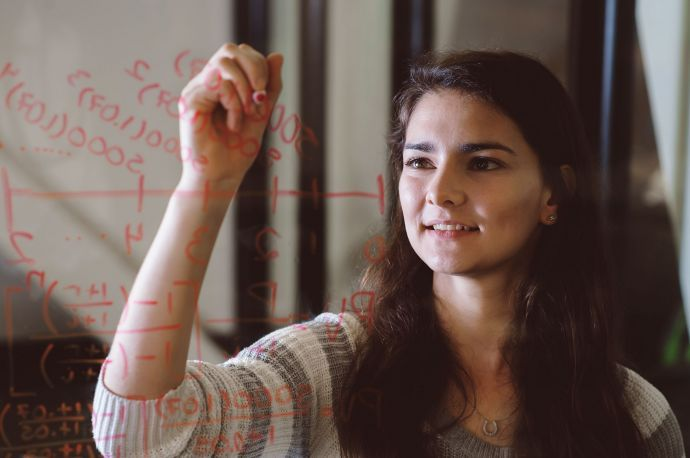The applied mathematics major is designed for students who are interested in applying their mathematics skills to problems in the real world, for example, in engineering. Our students graduate with a passion for mathematics and learning and are able to apply the problem-solving skills learned in the classroom to the world around them. Our mathematics program offers opportunities for you to refine your knowledge and use of mathematics content, communication and teamwork, mathematical reasoning and problem solving, and technology. Courses are intended to give you a competitive edge when you enter the job market.

Students who choose to major in mathematics are provided opportunities to develop strong problem solving skills that use quantitative methods and appropriate technology. They understand the strengths, limitations and wide applicability of mathematical modeling in a variety of disciplines. Students develop an appreciation for the discipline and esthetics of mathematics, effectiveness in problem solving, and an appropriate understanding of theory. Graduates who major in mathematics are prepared for the many careers in which mathematics plays an important role, for further study in Mathematics at the graduate level, or for careers in teaching mathematics.

The first course in all Mathematics majors is Calculus I, II or III depending on the student’s high school preparation in mathematics. Majors with AP Math AB or IB Math HL credit start in Calculus II. Majors with AP Math BC credit start in Calculus III. Students who are not able to start in Calculus I because of deficiencies in their algebra or Trig skills start in MATH 041, Precalculus. Students who place lower than MATH 041 discuss with their advisor how much extra time is required to complete their degree program because of the required developmental work. Mathematics majors are proficient with graphing calculators and consider taking elective courses that use quantitative skills in areas such as business, economics, computer science, science and engineering.

Minimum 46 units and 13 courses that include:

 MATH 051 Calculus I 4 MATH 053 Calculus II 4 MATH 055 Calculus III 4 MATH 075 Introduction to Linear Algebra 4 MATH 145 Applied Linear Algebra 4 COMP 051, or COMP 061 Introduction to Computer Science, or Introduction to Programming for Data Science 4 Select one of the following: 4 MATH 049 Introduction to Abstract Mathematics MATH 057 Applied Differential Equations I: ODEs Select three of the following (minimum 3 units per course): 9-12 MATH 037 Introduction to Statistics and Probability MATH 049 Introduction to Abstract Mathematics MATH 057 Applied Differential Equations I: ODEs MATH 072 Operations Research Models MATH 074 Discrete and Combinatorial Mathematics MATH 110 Numerical Analysis MATH 121 Financial Mathematics I MATH 122 Financial Mathematics II MATH 130 Topics in Applied Statistics MATH 131 Probability and Mathematical Statistics I MATH 132 Probability and Mathematical Statistics II MATH 133 Topics in Applied Statistics II MATH 148 Cryptography MATH 152 Vector Analysis MATH 157 Applied Differential Equations II MATH 174 Graph Theory MATH 193 Special Topics (to be approved by advisor) Three further electives chosen from a list of approved courses available in the mathematics department or math electives numbered MATH 049 or higher (excluding MATH 161, MATH 162, and MATH 166.) At most one elective may be numbered lower than 100. 9-12

Note: 1) Electives are to be chosen in consultation of a major advisor. 2) At most one elective may be numbered lower than 110. 3) One elective may be chosen from the following experiences: independent study, undergraduate research, internship, and practicum.